Skip to main content

# 5.7: 2D NMR Solutions

Exercise 5.1.1:

ethyl butanoate

Exercise 5.1.2:Exercise 5.1.3:Exercise 5.1.4:Exercise 5.1.5:Exercise 5.1.6: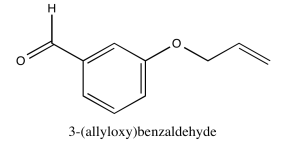Exercise 5.1.7:Exercise 5.1.8:Exercise 5.1.9:Exercise 5.1.10:Exercise 5.1.11: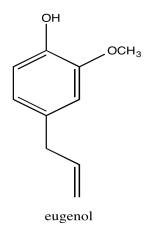Exercise 5.1.12:Exercise 5.2.1:Exericse 5.2.2: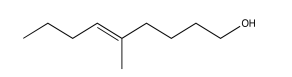Exercise 5.2.3:Exercise 5.3.1:Exercise 5.3.2:Exercise 5.3.3:Exercise 5.3.4:Exercise 5.3.5:Exercise 5.3.6:Exercise 5.3.7:Exercise 5.3.8:Exercise 5.4.1: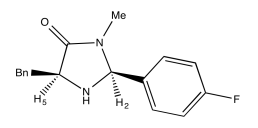Exercise 5.4.2:Exercise 5.4.3:

a) Due to resonance, there is substantial pi character to the amide bond which restricts free rotation around that bond.b)c)Exercise 5.4.4:Exercise 5.4.5:Exercise 5.4.6:

a)b)c)Exercise 5.4.7: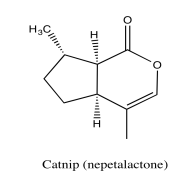Exercise 5.5.1:Exercise 5.5.2:Exercise 5.5.3: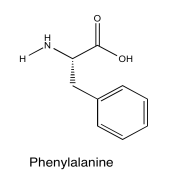Exercise 5.5.4:Exercise 5.5.5:Exercise 5.6.1:

1H NMR

 Chemical shift (ppm) Integration Multiplicity Partial Structure 5.54 2H multiplet CH=C (x 2) 4.17 2H doublet O-CH2-CH 2.08 2H quintet CH-CH2-CH3 1.47 1H boad singlet OH 0.95 3H triplet CH2-CH3

* total # H: 10

13C NMR

 Chemical shift (ppm) Type of carbon 134 sp2 128 sp2 58 sp3-O 21 sp3 14 sp3

*total # C: 5

COSY

 Assignment 1H COSY A 0.95 2.08 B 2.08 0.95, 5.54 C 4.17 5.54 D 5.54 2.08 E 5.54 4.17

*HMQC indicates two hydrogens at 5.54 are in two different environments

HMQC

 Assignment 13C 1H A 14 0.95 B 21 2.08 C 58 4.17 D 128 5.54 E 134 5.54

Formula:

C5H10O (1 O indicated from shift in 13C, 1H NMR)

FW = $$5 \times 12) + (10 \times 1) + (1 \times 16) = 86$$

Compare C5H10 ratio to C5H12 in hydrocarbon

Degrees of unsaturation = $$\frac{(2 \times 5) + 2 - 10}{2} = 1 unit (1 double bond) The data tables should be consitent with this structure: pent-2-en-1-ol (could be cis or trans based on this analysis) Exercise 5.6.2: 1H NMR:  Chemical shift (ppm) Integration Multiplicity Partial structure 4.7 5H singlet solvent 3.93 1H triplet CH2-CH-N 2.40 2H multiplet CH2-CH2? 2.09 2H multiplet CH2-CH2? 1.4 9H singlet C(CH3)3 *Total number of H: 19 H 13C NMR:  Chemical shift (ppm) Type of carbon 170 sp2 (C=O) 80 sp3 (C-O) 52 sp3 (C-N) 32 sp3 28 sp3 26 sp3 *Total number of C: 6 apparent, but two more suggested by symmetry (3 methyl groups in 1H NMR) for 8 C; a third extra suggested by MW fit for 9 C COSY:  Assignment 1H COSY Solvent 4.7 -- B 3.93 2.40 D 2.40 2.09 C 2.09 2.40, 2.09 A 1.4 -- Formula: C9H18O3N2 (extra O indicated from shift in 13C, 1H NMR; second O suggested by C=O in 13C NMR; additional CO needed to fit MW) FW = \((9 \times 12) + (18 \times 1) + (3 \times 16) + (2 \times 14) = 202$$

FW = ((9 \times 12) + (18 \times 1) + (3 \times 16) + (2 \times 14) = 202\)

Compare C9H18 to C9H22 for the corresponding hydrocarbon corrected for two nitrogens (therefore two extra hydrogens)

Degrees of unsaturation = $$\frac{(2 \times 9) + 2 + 2 - 10}{2} = 2$$ units (2 double bonds)

The data tables should be consistent with this structure:Exercise 5.6.3:

The data should be consistent with this structure: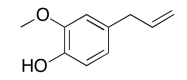Exercise 5.6.4:

1H NMR:

 Chemical shift (ppm) Integration Multiplicity Partial Structure 4.71 -- singlet solvent 4.17 1H doublet? CO-CH-N 3.75 3H singlet O-CH3 2.25 1H multiplet CH-CH-(CH3)2 0.92 6H triplet? 2 x CH3

13C NMR:

 Chemical shift (ppm) Type of carbon 170 sp2 C=O 60 sp3 C-N 52 sp3 C-O 30 sp3 C 19 sp3 C

COSY:

 Assignment 1H COSY A 4.17 2.25 B 3.75 -- C 2.25 4.17 D 0.92 2.25

Formula:

C6H13O2N (1 O indicated from shift in 13C, 1H NMR)

FW = $$(6 \times 120 + (13 \times 1) + (2 \times 16) + (1 \times 14) = 131$$

Degrees of unsaturation = $$\frac{(2 \times 6) + 2 + 1 - 13}{2} = 1$$ unit (1 double bond)

The data should be consistent with this structure:Exercise 5.6.5:

The data should be consistent with this structure:Exercise 5.6.6:

The data should be consistent with this structure:Exercise 5.6.7:

The data should be consistent with this structure:Exercise 5.6.8:

The data should be consistent with this structure:Exercise 5.6.9:

The data should be consistent with this structure:Exercise 5.6.10:

The data should be consistent with this structure:Exercise 5.6.11:

The data should be consistent with this structure:Exercise 5.6.12:

The data should be consistent with this structure:Exercise 5.6.13:

1H NMR:

 Chemical shift (ppm) Integration Multiplicity Partial structure 5.7 1H singlet C=CH-CO 2.77 1H multiplet CH2-CH-CO 2.62 1H multiplet C-CH-C 2.39 1H multiplet C-CH-C 2.05 1H doublet? C-CH-CH? 1.96 3H singlet C-CH3 1.48 3H singlet C-CH3 0.98 3H singlet C-CH3

13C NMR:

 Chemical shft (ppm) Type of carbon 204 sp2 C=O 170 sp2 121 sp2 59 sp3 55 sp3 50 sp3 41 sp3 28 sp3 24 sp3 22 sp3

COSY:

 Assignment 1H COSY 1 2.39 2.77, 2.62? 3 5.7 2.05? 5 2.62 2.62? 7a 2.77 2.05 7b 2.05 2.77 8 1.48 -- 9 0.98 -- 10 1.96 --

Formula:

C10H14O (1 O indicated from shift in 13C, 1H NMR)

FW = $$(10 \times 12) + (14 \times 1) + (1 \times 16) = 150$$

Degrees of unsaturation = \(\frac{(2 \times 10) + 2 - 14}{2} = 4 units (e.g. 2 rings, 2 double bonds)

The data should be consistent with this structure:Exercise 5.6.14:

The data should be consistent with this structure:Exercise 5.6.15:

The data should be consistent with this structure: